# HSPT Math : Concepts

## Example Questions

### Example Question #31 : Concepts

When evaluating the expression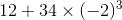,

in which order must the operations be carried out?

Explanation:

According to the order of operations, since no grouping symbols are present (the parentheses are setting apart a negative number, not an operation), the exponentiation (the cubing) is worked first, then the multiplication, then the addition.

### Example Question #31 : Concepts

When evaluating the expression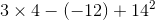,

which operation must be performed last?

Subtraction

Multiplication

Exponentiation

Explanation:

According to the order of operations, since no grouping symbols are present (the parentheses are setting apart a negative number, not an operation), the exponentiation must be performed first, followed by the multiplication. The remaining subtraction and addition are performed in left-to-right order, so the subtraction is worked next, and the addition is the final operation performed.

### Example Question #32 : Concepts

When evaluating the expression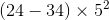,

in which order must the operations be carried out?

Multiplying, squaring, subtracting

Squaring, subtracting, multiplying

Subtracting, squaring, multiplying

Subtracting, multiplying, squaring

Subtracting, squaring, multiplying

Explanation:

According to the order of operations, the operation inside the parentheses, which is the subtraction, is performed first. This leaves a multiplication and an exponentiation (the squaring); by the order of operations, the squaring is performed next, then the multiplication.

### Example Question #31 : Concepts

Define an operation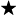on the real numbers as follows: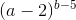Which of the following is an undefined quantity?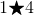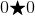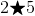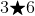Explanation:

If both the base and exponent of an exponential expression are integers, then the expression is undefined if and only if the base is 0 and the exponent is 0 or negative. Forto be undefined, it must hold that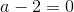, or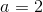.is the only possible choice; note that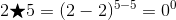, an undefined quantity.

### Example Question #33 : Concepts

When evaluating the expression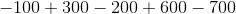,

which operation will be performed third?

The rightmost subtraction

The leftmost subtraction

Explanation:

In the order of operations, additions and subtractions are performed left to right. Therefore, the four operations are performed in the following order: leftmost addition, leftmost subtraction, rightmost addition, rightmost subtraction. Therefore, the rightmost addition is performed third.

### Example Question #35 : Arithmetic

Negative 37 is the square root of what number?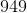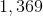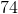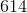Explanation:

The square root of a number is the number which, when squared - multiplied by itself - yields that number as its result. Recall that when two negative numbers are multiplied together they result in a positive numbers.

Therefore, negative 37 is the square root of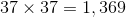### Example Question #34 : Concepts

Which of the following four expressions is the greatest?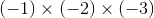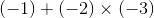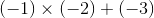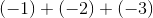Explanation:

By order of operations, multiplication takes precedence over addition, and each is worked right to left. Keeping this in mind: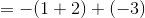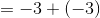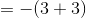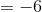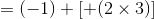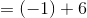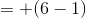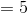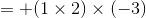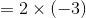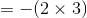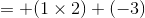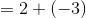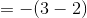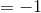is the greatest of the four.

### Example Question #38 : Concepts

Which of the following is the least of the four expressions?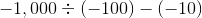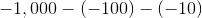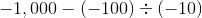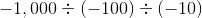Explanation:

By order of operations, division takes precedence over subtraction, and each is worked right to left. Keeping this in mind: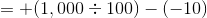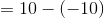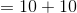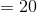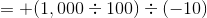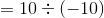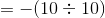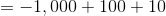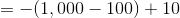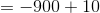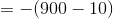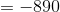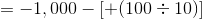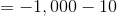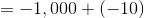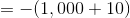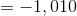is the least of the four.

### Example Question #34 : Arithmetic

Solve: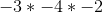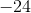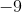Explanation:

Whenever you multiple an odd number of negative values togther, you will end with a negative number.

Then you just need to multiple your actual values together.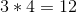and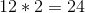so your final answer is.

### Example Question #35 : Concepts

Which of the following is a negative number?

(I)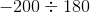(II)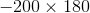(III)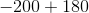(II) only

All three

(I) only

(III) only